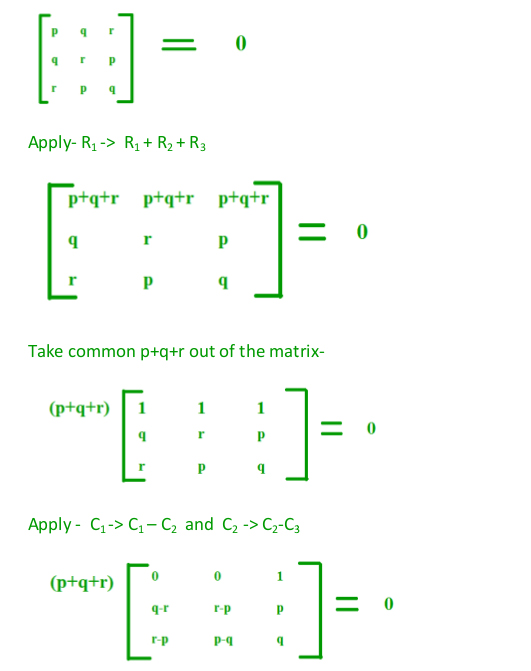# GATE | GATE-CS-2015 (Set 3) | Question 43

If the following system has non-trivial solution,

```  px + qy + rz = 0
qx + ry + pz = 0
rx + py + qz = 0 ```

then which one of the following options is True?

(A) p – q + r = 0 or p = q = –r
(B) p + q – r = 0 or p = –q = r
(C) p + q + r = 0 or p = q = r
(D) p – q + r = 0 or p = –q = –r

Explanation: For non-trivial solution, |A| should be equal to 0
Hence,Now solve it using matrix rules:

(p+q+r) [(q-r)(p-q) – (r-p) (r-p) ] = 0

Either (p+q+r) = 0 or [(q-r)(p-q) – (r-p) (r-p) = 0

From (p+q+r) =0, you can clearly say that option C is the correct one.

and for more precise answer, let’s solve second equation:

[(q-r)(p-q) – (r-p) (r-p) = 0

(q-r)(p-q) = (r-p) (r-p)

and only p = q = r satisfies this equation. So option C is correct one.

This explanation has been contributed by Nitika Bansal.

Quiz of this Question

My Personal Notes arrow_drop_up
Article Tags :

Be the First to upvote.

Please write to us at contribute@geeksforgeeks.org to report any issue with the above content.